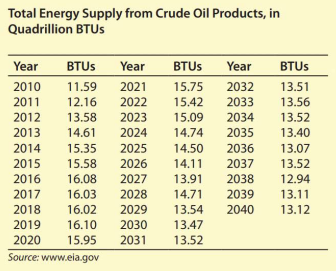# II. Energy from Crude Oil (Modeling) The table shows the total energy supply from crude oil products, in quadrillion BTUs, for the years from 2010 and projected through 2040. Find the cubic function that models the data, with x equal to the number of years past 2010 and y equal to the number of quadrillion BTUs of energy. Report the model as y = f ( x ) with three significant digit coefficients.### Mathematical Applications for the ...

11th Edition
Ronald J. Harshbarger + 1 other
Publisher: Cengage Learning
ISBN: 9781305108042

#### Solutions

Chapter
Section### Mathematical Applications for the ...

11th Edition
Ronald J. Harshbarger + 1 other
Publisher: Cengage Learning
ISBN: 9781305108042
Chapter 9, Problem 1EAGP2
Textbook Problem
1 views

## II. Energy from Crude Oil (Modeling)The table shows the total energy supply from crude oil products, in quadrillion BTUs, for the years from 2010 and projected through 2040.Find the cubic function that models the data, with x equal to the number of years past 2010 and y equal to the number of quadrillion BTUs of energy. Report the model as y = f ( x ) with three significant digit coefficients.

This textbook solution is under construction.

### Still sussing out bartleby?

Check out a sample textbook solution.

See a sample solution

#### The Solution to Your Study Problems

Bartleby provides explanations to thousands of textbook problems written by our experts, many with advanced degrees!

Get Started